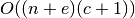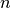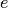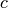Warning

This documents an unmaintained version of NetworkX. Please upgrade to a maintained version and see the current NetworkX documentation.

# simple_cycles¶

simple_cycles(G)[source]

Find simple cycles (elementary circuits) of a directed graph.

An simple cycle, or elementary circuit, is a closed path where no node appears twice, except that the first and last node are the same. Two elementary circuits are distinct if they are not cyclic permutations of each other.

This is a nonrecursive, iterator/generator version of Johnson’s algorithm . There may be better algorithms for some cases  .

Parameters: G (NetworkX DiGraph) – A directed graph cycle_generator – A generator that produces elementary cycles of the graph. Each cycle is a list of nodes with the first and last nodes being the same. generator

Examples

>>> G = nx.DiGraph([(0, 0), (0, 1), (0, 2), (1, 2), (2, 0), (2, 1), (2, 2)])
>>> len(list(nx.simple_cycles(G)))
5


To filter the cycles so that they don’t include certain nodes or edges, copy your graph and eliminate those nodes or edges before calling

>>> copyG = G.copy()
>>> copyG.remove_nodes_from()
>>> copyG.remove_edges_from([(0, 1)])
>>> len(list(nx.simple_cycles(copyG)))
3


Notes

The implementation follows pp. 79-80 in .

The time complexity isfornodes,edges andelementary circuits.

References

  (1, 2) Finding all the elementary circuits of a directed graph. D. B. Johnson, SIAM Journal on Computing 4, no. 1, 77-84, 1975. http://dx.doi.org/10.1137/0204007
  Enumerating the cycles of a digraph: a new preprocessing strategy. G. Loizou and P. Thanish, Information Sciences, v. 27, 163-182, 1982.
  A search strategy for the elementary cycles of a directed graph. J.L. Szwarcfiter and P.E. Lauer, BIT NUMERICAL MATHEMATICS, v. 16, no. 2, 192-204, 1976.